# iFit: Models (fit models)

### How to use models

Models are numerical functions, that take input axes and a parameter set, and return a function evaluation.

Such models are defined as iFunc objects, and used in iFit when there is a need to e.g. compute and plot a function evaluation corresponding with the axes of an iData object. Similarly, this function evaluation can be used iteratively in an optimization process in order to find a parameter set that matches best the iData object Signal, using the fits method (refer to the Fit page).

Models can be used in the following ways (for instance 'model' may be 'gauss'):
`>> model		% display model information>> disp(model)		% idem, extensive information>> plot(model)		% plot the model with its default settings>> model(p, x, y ...)	% evaluate the model with parameters p, and axes x,y,...>> model([], x, y ...)	% evaluate the model with axes x,y,... and automatic parameter guess>> model('guess', x, y ...)	% idem>> a(model, p)		% evaluate the model onto the iData object 'a' axes with parameters p>> fits(a, model, p)	% fit the model onto the iData object 'a'>> fits(model, a, p)	% same as above>> save(model, 'filename', 'mat') % save the Model as a Matlab file. orher formats are possible (YAML, m, JSON, XML...)>> iFunc('filename')	% import a Model stored as m, YAML, MAT, JSON file format.`
To create a model, use the ifitmakefunc dialogue window (see below), or instantiate an iFunc object.
`>> model = gauss;>> model = gauss+lorz;	% add models>> model = iFunc('p(1)*x+p(2)')>> model = iFunc('a=p(1); b=p(2); signal=a*x+b') `
You can edit their code to see how to define new models:
`>> edit gauss % edit the function definition (from a file)>> edit voigt>> edit(voig) % edit the object definition`
It is also possible to create a model out of an iData (data set) object. Then, the model parameters are the total intensity scaling, as well as an offset and a scaling factor per axis. In order to create a model from a data file, use:
`	>> iFunc(iData('filename'))`
will create a data set object and derive a model out of it.

### List of default fit models

The 1D functions are signal=f(parameters, x), and the 2D functions are signal=f(parameters, x, y).

 Function Description Dimensionality Parameters allometric Allometric (power/asymptotic law) 1D Amplitude Offset Exponent BackGround bigauss Asymmetric Gaussian 1D Amplitude Centre HalfWidth1 HalfWidth2 Background bilorz Asymmetric Lorentzian 1D Amplitude Centre HalfWidth1 HalfWidth2 Background bose Bose factor 1D Tau [h/2pi/kT] in "x" units dho Damped harmonic oscillator 1D Amplitude Centre HalfWidth Background Temperature (in "x" unit) dirac Dirac peak 1D Amplitude Centre doseresp Dose-response curve with variable Hill slope. This is a sigmoid or S-shaped. 1D Amplitude Center Slope BackGround expon Exponential decay 1DAmplitude Tau Background expstretched Exponential - Stretched 1D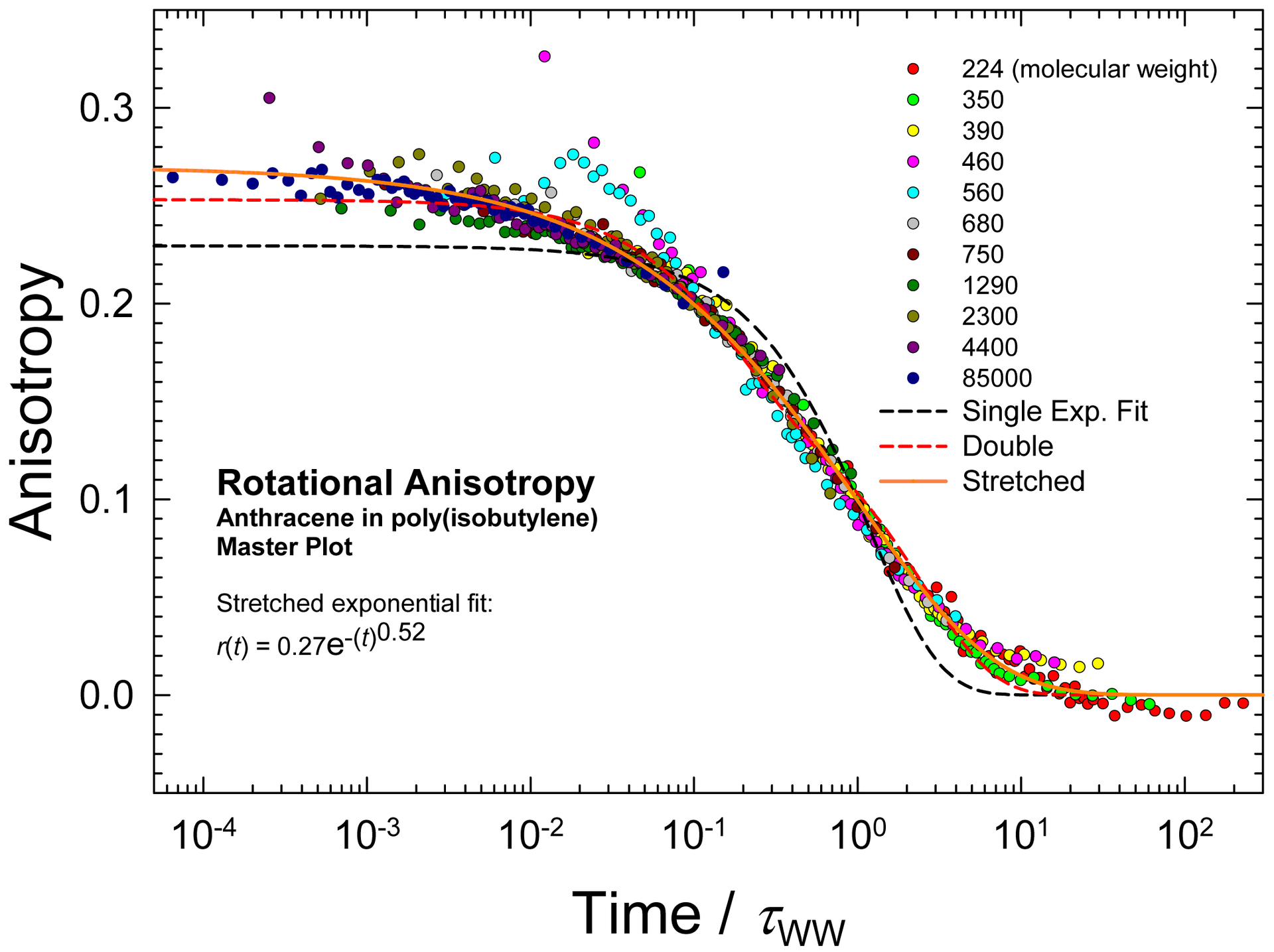Amplitude Tau Exponent Background gauss Gaussian where the HalfWidth is in fact σ. The 'true' half width is thus 1.177*HalfWidth. 1D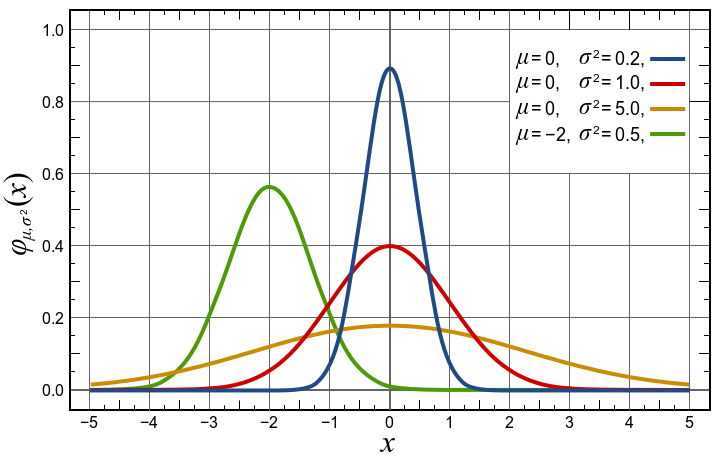Amplitude Centre HalfWidth Background green Green function 1D Amplitude Centre HalfWidth Background heaviside Heaviside (gap) The GapSide indicates raising (+) or falling (-) gap. 1D Amplitude Centre GapSide Background langevin Langevin function for magnetic polarization 1D Amplitude Center Width BackGround laplace Laplace distribution function 1D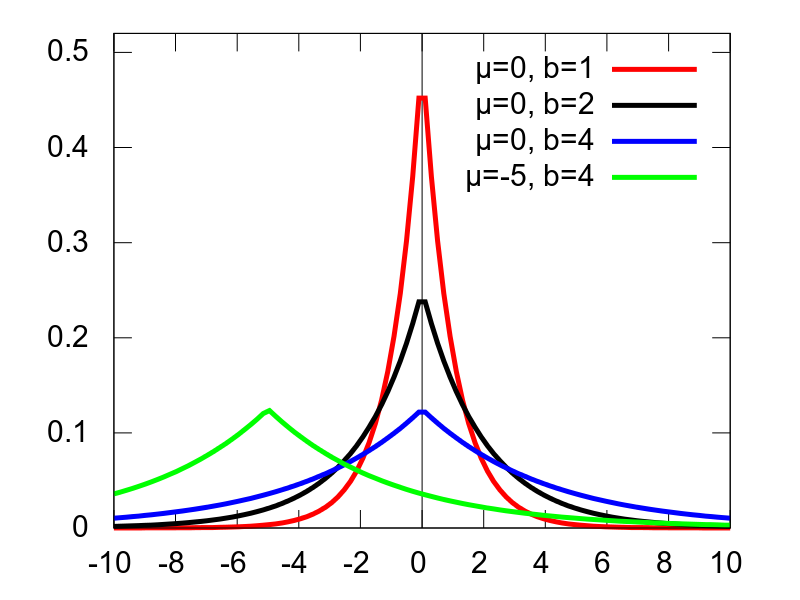Amplitude Center Width BackGround lognormal Log-Normal distribution 1D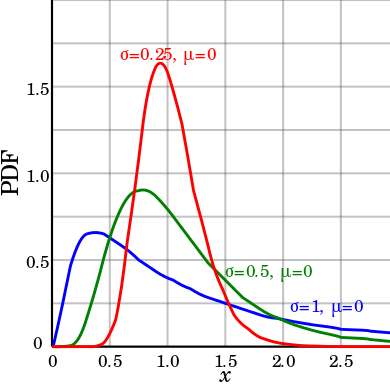Amplitude Center Width BackGround lorz Lorentzian (aka Cauchy) used with Amplitude uncorrelated to Width. 1D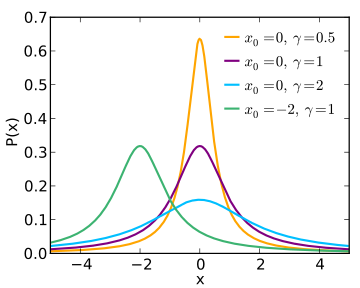Amplitude Centre HalfWidth Background ngauss multiple Gaussian where the HalfWidth is in fact σ. The 'true' half width is thus 1.177*HalfWidth. n*1D nlorz multiple Lorentzian used with Amplitude uncorrelated to Width. n*1D pareto Pareto distribution function 1D Amplitude Exponent Width BackGround poisson Poisson distribution WARNING: The 'x' axis is assumed to be an integer array (counts) 1D Amplitude Center BackGround pseudovoigt Pseudo Voigt 1D Amplitude Center Width BackGround LorentzianRatio quadline Quadratic line (parabola) 1DQuadratic Linear Constant sigmoid Sigmoidal S-shaped curve (similar to Dose Response) 1D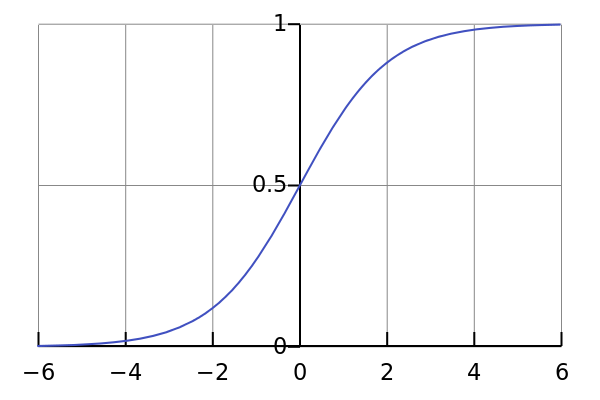Amplitude Center Width BackGround sine Sine function 1D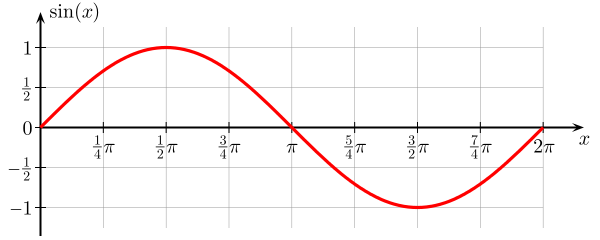Amplitude Phase_Shift Period BackGround sinedamp Damped Sine function (exponential decay) 1DAmplitude Phase_Shift Period BackGround Decay strline Straight line 1D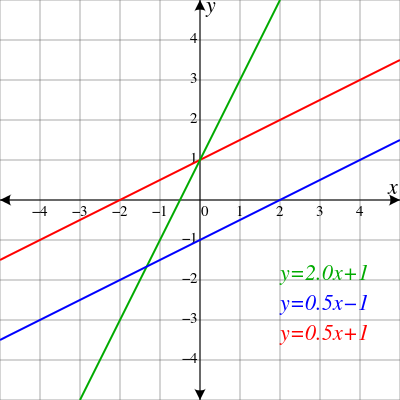Gradient Background triangl Triangular 1D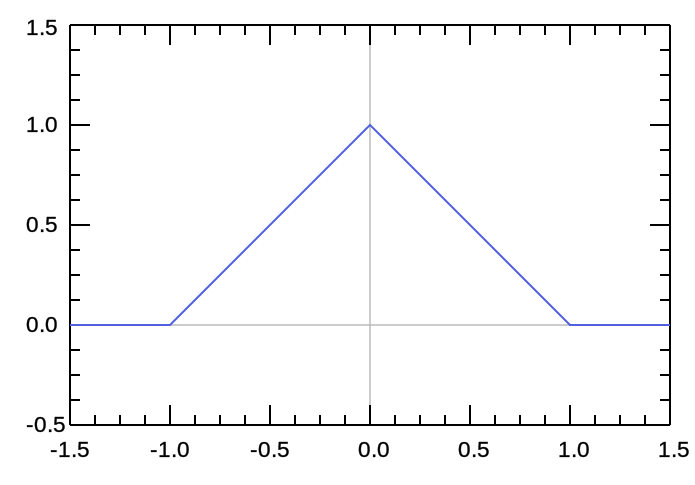Amplitude Centre HalfWidth Background tophat Top-Hat rectangular function 1D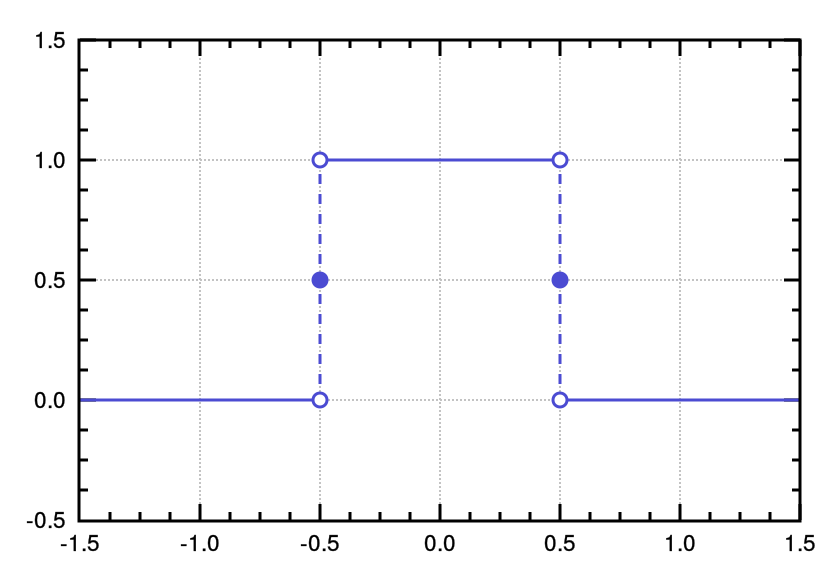Amplitude Centre HalfWidth Background twoexp Two exponential decay functions 1D Amplitude1 Tau1 Amplitude2 Tau2 Background voigt Voigt function 1D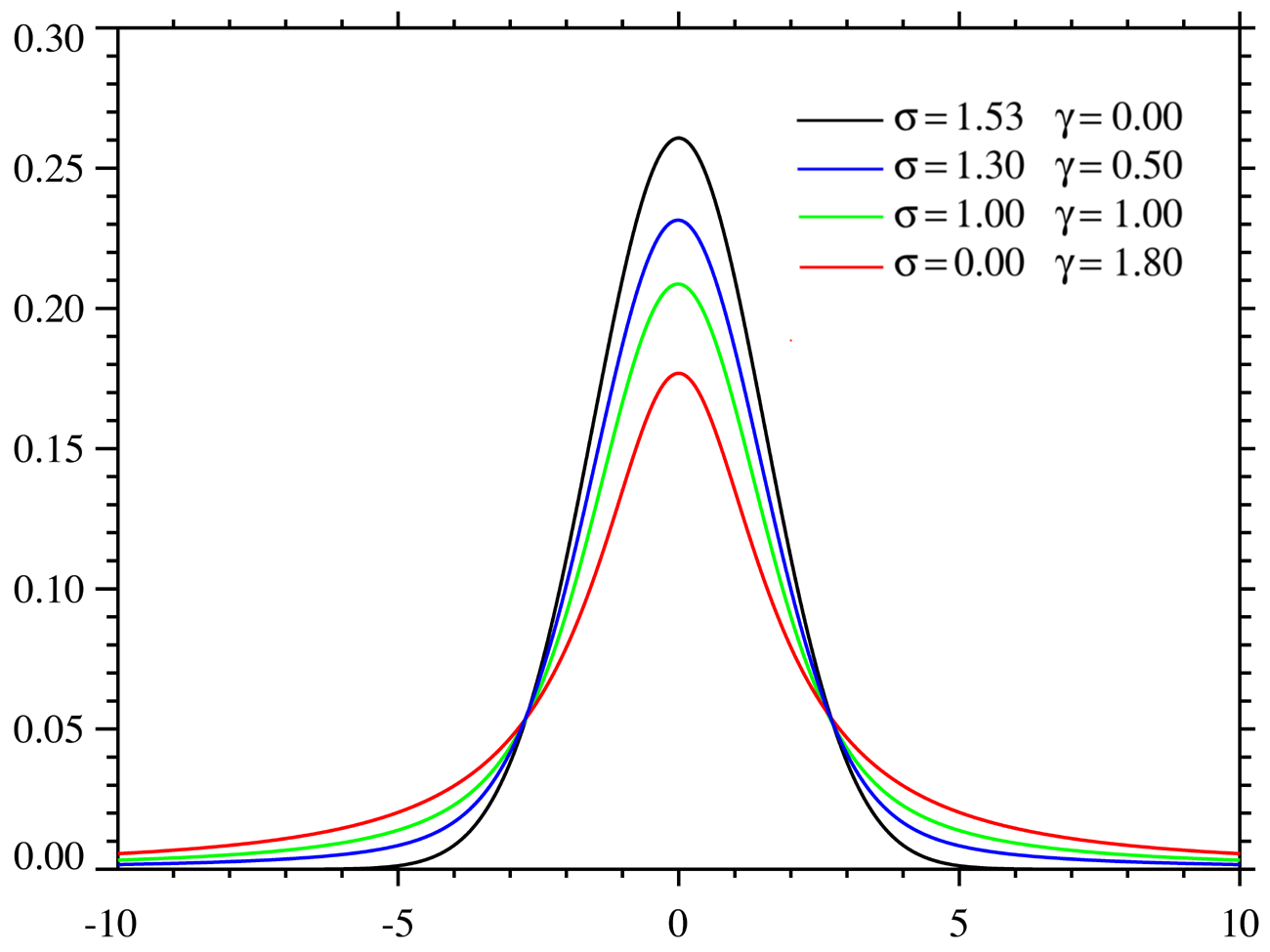Amplitude Centre HalfWidth_Gauss HalfWidth_Lorz Background gauss2d Gaussian function with tilt angle where the HalfWidth is in fact σ. The 'true' half width is thus 1.177*HalfWidth. 2D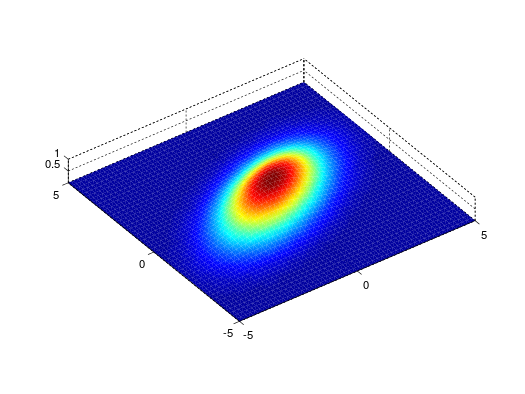Amplitude Centre_X Center_Y HalfWidth_X HalfWidth_Y Angle Background lorz2d Lorentzian function with tilt angle used with Amplitude uncorrelated to Width. 2D Amplitude Centre_X Center_Y HalfWidth_X HalfWidth_Y Angle Background plane2d Planar function 2D Slope_X Slope_Y Background pseudovoigt2d Pseudo Voigt with tilt angle 2DAmplitude Centre_X Center_Y HalfWidth_X HalfWidth_Y Angle Background LorentzianRatio quad2d Quadratic (parabola) with tilt angle 2D Amplitude Centre_X Center_Y Curvature_X Curvature_Y Angle Background gaussnd n-dimensional Gaussian nD sf_hard_spheres Hard Sphere structure factor [Percus-Yevick] 1D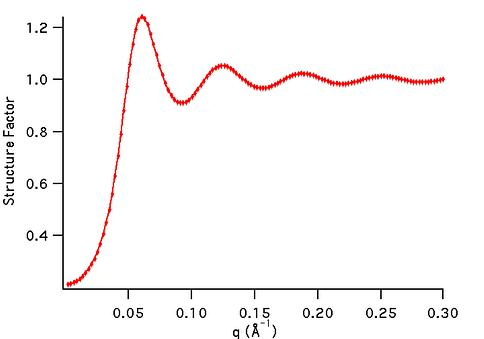R rho rietveld Rietveld refinement of powder sample with full McStas instrument model 1D, 2D, 3D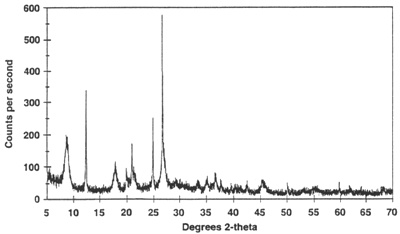sample structure, instrument parameters sqw_sine3d Phonon dispersions as sine wave in HKL with a damped harmonic oscillator energy dispersion 4D (HKLw)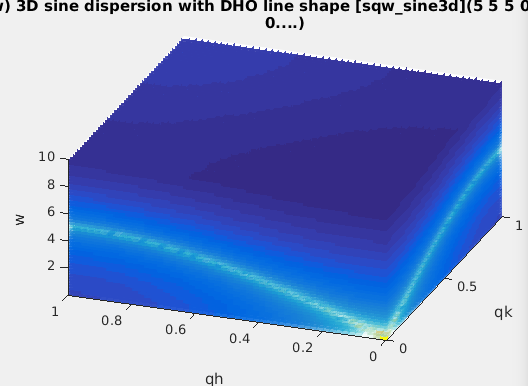zone center, energy gaps, periodicity, DHO width, temperature, background sqw_spinw Spin-wave dispersion in HKL using SpinW. 4D (HKLw)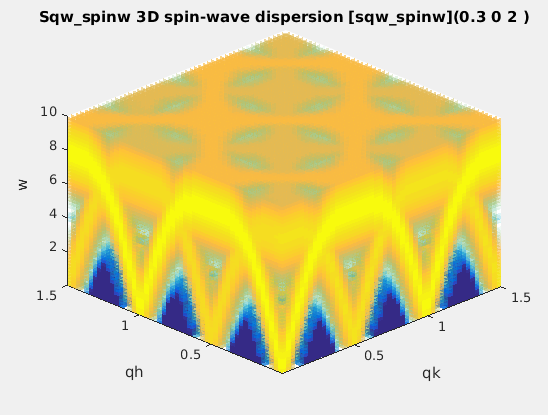energy broadening, Temperature, Amplitude, coupling parameters J... sqw_vaks Phonon dispersions in perovskite cubic crystals using the Vaks parameterisation 4D (HKLw)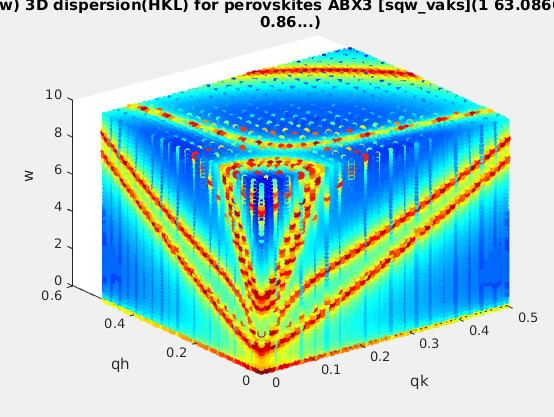acoustic and optical energies, coupling parameters, soft mode frequency, DHO width, temperature, background sqw_cubic_monoatomic Phonon dispersions in a monoatomic cubic crystal using the Dynamical matrix. 4D (HKLw)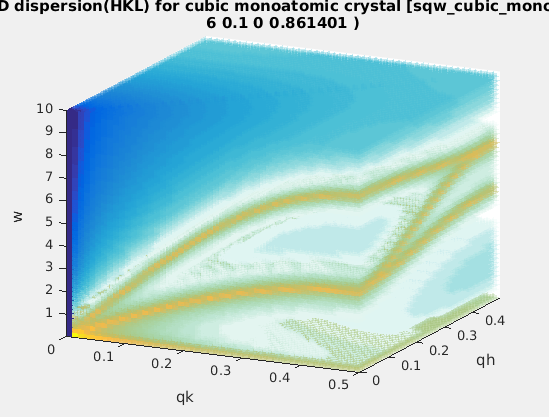acoustic force constant ratio and energy scaling, DHO width, temperature, background sqw_phonons Phonon dispersions from the Dynamical matrix, using forces estimated by ab-initio DFT using ASE 4D (HKLw)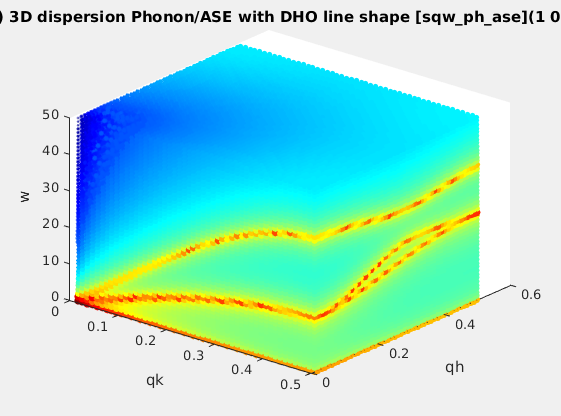Creation: POSCAR, CIF, or PDB, ... Then, only the DHO line shape. ab-initio implies no (few) tunable parameter. sqw_linquad A phenomenological dispersion which can describe e.g. an acoustic/linear mode, with quadratic expansion in other directions. This model can be considered as a local expansion in series of any dispersion. 4D (HKLw)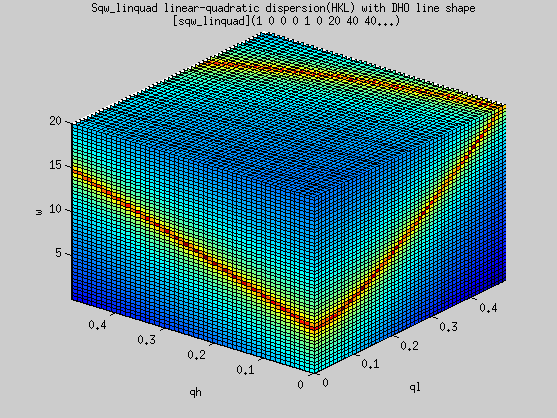Energy and location of 'gap', slopes, directions of slopes, DHO width, temperature, background sqw_acoustopt A phenomenological dispersion which can describe e.g. a pure acoustic or optical mode, with quadratic expansion around a minimum. 4D (HKLw)Energy and location of 'gap', slopes, directions of slopes, DHO width, temperature, background sqw_spinwave Spin-wave dispersion in HKL using SPINWAVE (LLB). 4D (HKLw) Amplitude, and user defined parameters (coupling...)

To get one of these models, just type their function name:
`>> gauss>> a = gauss;`
The list of all available fit functions can be obtained from the command:
`>> fits(iData);`
which also produces the plot above.

### Specialized models

#### Small Angle Scattering models (neutrons, x-rays)

We list below a number of models used to describe neutron and x-ray scattering from matter. The structure factor S(q) accounts for the structure of matter at the atomistic/molecular level, whereas the form factor P(q) accounts for the geometrical arrangement of large scale scattering units (micelles, tubes, ...). In practice, the scattering from a material can be described by:

I(q) = P(q).S(q)

where q is the momentum exchange in the material. These models have been extracted from:

 Structure factors Description Dimensionality Parameters sf_hard_spheres Hard Sphere structure factor [Percus-Yevick] 1DR rho sf_square_well structure factor of particles interacting with a square well potential [Sharma] 1D R rho epsilon Delta T sf_sticky_hard_spheres Sticky Hard Sphere structure factor [Baxter/Menon] 1D R rho tau

 Form factors: small angle Description Dimensionality Parameters ff_core_shell Spherical/core shell form factor [Guinier] 1D R1 R2 eta1 eta2 ff_sphere Sphere form factor [Guinier] 1D R eta

#### Powder structure refinement (Rietveld)

The rietveld model performs a structure refinement (atom type and position, structure group) of a powder by comparing a measured diffractogram with a simulated diffractogram using McStas and CrysFML. This model requires external software to be installed on your computer. See Requirements below.

 Powder diffraction Description Dimensionality Parameters rietveld Rietveld refinement of powder sample with full McStas instrument model 1D, 2D, 3Dsample structure, instrument parameters
The 'rietveld' model allows to prepare a sample+instrument model in order to fit a diffraction/structure data set.
It is obtained by making use of the CrysFML library:
The procedure is to import a crystal structure (CIF, ShelX, FullProf/CFL format) then compute the HKL d-F2 list of reflections (for crystal or powder), and insert this powder model into a diffractometer simulated using McStas. The detector image, which is the convolution of the sample structure with the instrument resolution, is then compared with a given diffractogram, and optimised to refine the crystal parameters.

The syntax to build the model, and then perform the refinement is:
`>> model=rietveld(structure, ...., instrument, ....)>> p = fits(model, measurement);`
where the 'structure' describes the initial sample structure (space group, cell parameters abc αβγ, atom positions, ...); it can be given as a CIF, FullProf/CFL or ShelX file, as well as from a struct type variable with fields 'Spgr' and 'cell', followed with atoms (giving xyz Biso occupancy spin charge, where only xyz are mandatory, others are optional):
```Sample.cell  = [10.242696  10.242696  10.242696  90.000  90.000  90.000];
Sample.Spgr  = 'I 21 3';
Sample.Ca1   = [0.46737  0.00000  0.25000  0.60046  0.50000   0.0   2.0];...```
the instrument is the name of the McStas instrument description (templateDIFF.instr is the default). The McStas simulation can be controlled using the default McStas options, as described in the dedicated help page : dir, ncount, mpi, seed, gravitation, compile, monitors. The monitors options is particularly recommended to specify which monitor file to use from the virtual experiment. The resulting model data set should be of the same type as the diffractometer measured data set (e.g. TOF-angle, height-angle, ...), but not necessarily with same binning and axes range.

Any other 'name=value' argument is assumed to be an instrument parameter. Any scalar parameter is made into a model parameter, whereas string/char parameters are set as fixed instrument parameters. By default, the sample structure (powder/single crystal) is made available to the McStas instrument by mean of a 'reflections.laz' file which should be used by a PowderN or Isotropic_Sqw component. This name can be changed by specifying the 'CFML_write=filename' option.

An example of Rietveld model is obtained from a CFL file, and the templateDIFF instrument including PowderN(reflections=Powder,...). We thus specify the fixed instrument parameter Powder='reflections.laz'. The wavelength is kept fixed at 2.36, and we use monitor names matching BananaTheta.
`>> model = rietveld([ ifitpath 'Data/Na2Ca3Al2F14.cfl' ], 'templateDIFF.instr', 'Powder=reflections.laz; lambda="2.36"; monitors=BananaTheta');`
Once built, it is possible to set constraints on the model with the syntax such as (see iFunc page) :
```>> model.parameter='fix'     % to lock its value during a fit process>> model.parameter='clear'   % to unlock value during a fit process
>> model.parameter=[min max] % to bound value>> model.parameter=[nan nan] % to remove bound constraint>> model.parameter=''        % to remove all constraints on 'parameter'>> model.Constraint=''       % to remove all constraints```
Then we import a data set
`>> measurement = iData([ ifitpath 'Data/nac_1645179.dat' ]);`
The refinement is then obtained by starting :
`>> parameters = fits(model, measurement)`
with optional arguments as described in the Fit page (the 'constraints' argument of fits is partly redundant with the model constraints seen above).
Remember to bound most or all of the model parameters for a faster and more reliable convergence of the optimization.

You may look at the following resources to get material structure files:

References:
1. CrysFML, Commission on Crystallographic Computing, IUCr Newsletter No.1, pp 50-58,  January 2003 [link].
2. McStas: K. Lefmann and K. Nielsen, Neutron News 10, 20, (1999) ; P. Willendrup, E. Farhi and K. Lefmann, Physica B, 350 (2004) 735.

Requirements/installation:
McStas must be installed. Packages exist for all major systems (Linux as RedHat and Debian flavours, Windows, Mac OSX). For Debian-class systems:
```cd /etc/apt/sources.list.d
sudo wget http://packages.mccode.org/debian/mccode.list
sudo apt-get updatesudo apt-get install mcstas-suite```
The CrysFML does not need to be installed, as the used bits are assembled in the cif2hkl fortran programme, which generates HKL d-F2 reflection lists suitable for the PowderN and Isotropic_Sqw McStas components. The cif2hkl programme is part of the iFit distribution, and is compiled by calling the local fortran compiler (gfortran) on demand.

#### Phonon/spin-wave dispersion (simple)

The sqw_sine3d model provides a simple way to model phonon-type dispersions, including simple spin-waves, acoustic and optical modes, incommensurate dispersions.

Limitation: this model only handles simple sine dispersions, and can not treat mode exchange (interferences). For more advanced spin-wave models, use the sqw_spinwave Model (below).

 S(q,w) Description Dimensionality Parameters sqw_sine3d Phonon dispersions as sine wave in HKL (3D) with a damped harmonic oscillator energy dispersion 4D (HKLw)zone center, energy gaps, periodicity. Axes are in rlu.

Each dispersion is a sine wave which goes continuously from energy E0 to E1, along 3 principal lattice directions (HKL). The dispersion has an energy width (DHO). Schematically, the dispersion relation is:

w(Q) = E0 + (E1-E0)*sin(Q_freq*pi*(Q-Q0));         along principal axes

where the wave-vector/momentum Q is expressed in reciprocal lattice units [r.l.u]. The parameters of this model allow extended flexibility in the description of the mode. Along the 3D HKL volume, a dispersion is described with 10 parameters.
The Q_freq parameter indicates how many dispersion sine 'arches' there are per reciprocal lattice unit [rlu]. A Q_freq of 1/2 means the dispersion extends from e.g. Q=0 to Q=2 rlu. A Q_freq of 1 means it extends from Q=0 to Q=1 rlu, and a Q_freq of 2 means there are two arches between Q=0 and Q=1 rlu (all these with Q0=0). A Q_freq of 0 sets a flat dispersion.

To create the model without defined parameter values, you may use:

sw = sqw_sine3d;

or alternatively, to define starting parameters:
sw = sqw_sine3d(p);
where p=[...] is a vector containing the parameter values. It may be given as 1,2,3 and 14 value vector, as detailed below.

A spin-wave could for instance mostly use Q0=0, Q_freq=1,  E0=0, E1>0 (2 arches from Q=0 to 1 rlu). In a simple anti-ferromagnet, the gap width is E1-E0=4J.S with J=exchange energy and S=magnetic moment of spins.

`  >> sw = sqw_sine3d([ E0 E1 Q_freq ])	% creates a dispersion from E0 to E1 with given Q frequency, e.g. .5, 1 or 2`

A phonon acoustic branch could use Q0=0, Q_freq=.5, E0=0 (1 arch from Q=0 to 1 rlu).

`  >> acoustic = sqw_sine3d(Emax)	% creates an acoustic dispersion up to Emax `

An phonon optical branch could use Q0=0, Q_freq=.2, E0>E1 E1>0 (1 arch from Q=0 to 1 rlu with Q=0 energy - Raman frequency).

`  >> optical = sqw_sine3d([ E0 E1 ])	% creates an optical dispersion from E0 to E1`

The model parameters allow to tune the dispersion:

• To shift the minimum/maximum Q of the dispersion, move QH0,QK0,QL0 parameters.
• To change the extent of the dispersion in Q, vary QH_freq,QK_freq,QL_freq parameters.
• To change to minimum and maximum energy, move E0 and E1_qh,E1_qk,E1_ql parameters.
• To model an incommensurate dispersion, move both QH0,QK0,QL0 and the QH_freq,QL_freq,QK_freq parameters to incommensurate (non rational) values.
• An anisotropic model can be obtained by having different E1 and Q_freq parameters along axes.
• A 1D or 2D dispersion can be obtained when setting the frequencies (e.g. QK_freq QL_freq) to 0

The model parameters are:

p(  1)=               E1_qh energy at QH half period [meV]
p(  2)=               E1_qk energy at QK half period [meV]
p(  3)=               E1_ql energy at QL half period [meV]
p(  4)=                  E0 zone-centre energy gap [meV]
p(  5)=                 QH0 QH zone-centre [rlu]
p(  6)=                 QK0 QK zone-centre [rlu]
p(  7)=                 QL0 QL zone-centre [rlu]
p(  8)=             QH_freq QH frequency [multiples of pi]
p(  9)=             QK_freq QK frequency [multiples of pi]
p( 10)=             QL_freq QL frequency [multiples of pi]
p( 11)=               Gamma Damped Harmonic Oscillator width in energy [meV]
p( 12)=         Temperature [K]
p( 13)=           Amplitude
p( 14)=          Background

The axes needed for the evaluation are expressed in rlu for QH,QK,QL and in meV for the energy.
The Model evaluation is as usual (in 4D):

model(p, qh, qk, ql, w)    % return a matrix. p can be [] to use guessed/default parameters
iData(model, p, qh, qk, ql, w)    % return an iData.

A usage example is as follows:`>> ac=sqw_sine3d(5); 		% an acoustic branch up to 5 meV>> qh=linspace(0,1,50);qk=qh; ql=qh'; w=linspace(0.01,10,50);	% the axes for evaluation>> f=iData(ac,[],qh,qk,ql,w);	% evaluate the model onto given axes>> plot3(log(f(:,:,1,:))); 	% plot as volume in [QH,QK,w, QL=0]. You can also use surf and scatter3 for other rendering`

The axes are given as vectors, but the second is made non-parallel to the others, to indicate we wish to build a volume out of this. Without transposing the vector, as all axes are the same length, they would be interpreted as event data, and the resulting evaluation would only contain 50 values.

It is possible to stack as many modes as possible, in different flavors. In this case it is advisable to link e.g. the Temperature and Background parameters: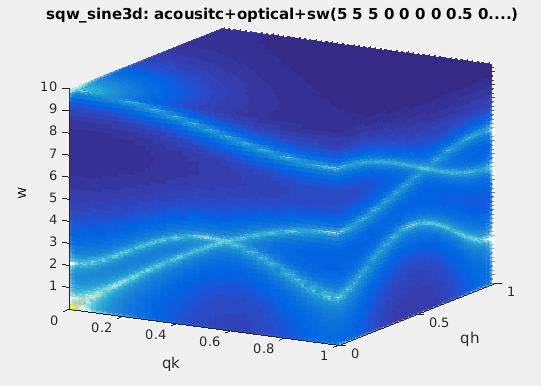`>> acoustic = sqw_sine3d(5); optical = sqw_sine3d([ 10 8 ]); sw = sqw_sine3d([ 2 4 1 ]);>> disp3 = acoustic + optical + sw;>> disp3.Temperature_2 = '"Temperature"';		% Temperature_2 = Temperature (1st sin3d)>> disp3.Temperature_3 = '"Temperature"';		% Temperature_3 = Temperature>> disp3.Name='sqw_sine3d: acousitc+optical+sw';>> mlock(disp3, {'Background_2','Background_3'});	% keep them as 0 (default)>> qh=linspace(0,1,50);qk=qh; ql=qh; w=linspace(0.01,10,51);	% the axes for evaluation>> f=iData(disp3,[],qh,qk,ql,w);			% evaluate using initial model parameters>> plot3(log(f(:,:,1,:))); 				% plot as volume in [QH,QK,w, QL=0].`

The plot (surf), plot3, scatter3, and slice methods for plotting all provide nice looking rendering of volume data. See Plot/3D page.

#### Spin-wave model (SpinW)

The sqw_spinw model can embed a spin-wave model from the SpinW to " simulate magnetic structures and excitations of given spin Hamiltonian using classical Monte Carlo simulation and linear spin wave theory."
Basically, SpinW allows to model a crystal structure including a spin Hamiltonian in the form:

H = ij SiJijSji SiAiSi + B i giSi

where S are the spin vectors, J are the spin-spin coupling matrices, A are the spin anisotropy matrices, B is the external magnetic field, and g is the g-tensor.

 S(q,w) Description Dimensionality Parameters sqw_spinwSpin-wave dispersion in HKL using SpinW. 4D (HKLw)energy broadening, Temperature, Amplitude,  parameters J... Axes are in rlu.

A SpinW object must first be created, and is then converted into an iFunc object using the syntax:
s = sqw_spinw(sw);
or
s = iFunc(sw);

The SpinW toolbox allows to build spin-wave models incuding spin-spin pair coupling, anisotropy terms and external field contribution. The model is then converted into an iFunc object, so that fitting and plotting is straightforward. An easy way to build a SpinW object is to read a CIF file, with syntax:

s = sqw_spinw(sw('cif_file'))

Additional options can be used when creating the Model, with:

s = sqw_spinw(sw, options);

where 'options' is a structure that can hold:
• options.component: a string to specify the component to use as intensity, as documented in sw_egrid function. Default is 'Sperp'. Suggested is also 'Sxx+Syy+Szz'.
Once created, the model can be evaluated and used for fitting.
The model parameters are:
p(1)=            Gamma       energy broadening (instrumental) [meV]
p(2)=            Temperature of the material [K]
p(3)=            Amplitude
p(4...)=         coupling parameters of the Hamiltonian (J)
The axes needed for the evaluation are expressed in rlu for QH,QK,QL and in meV for the energy [1 meV = 241.8 GHz = 11.604 K = 0.0965 kJ/mol]. The model can be evaluated by giving axes and model parameters (see examples below).
• When all axes are given as vectors of same orientation and same length, the HKLE set is assumed to be list of 'events'.
• When the H K L axes are vectors of same length/orientation, the HKL locations is assumed to be a q-path in the BZ, but the E (energy) axis can be of different length/orientation to compute the Model along (q,E) path, resulting in a 2D object. Typically, the band_structure function does this, to provide the phonon dispersion curve along principal directions.
• When the H K L E axes are given as mixed orientations/length, as vectors or matrices, the Model evaluation is done on a HKLE cube, which is well suited to visualize the whole dispersion surfaces. In the example below, ql has a different orientation (it is transposed) as qh and qk, which triggers a 4D HKLE cube evaluation.
The Model evaluation is as usual (in 4D):
model(p, qh, qk, ql, w)    % return a matrix. p can be [] to use guessed/default parameters
iData(model, p, qh, qk, ql, w)    % return an iData
As an example, we plot a simple square lattice Heisenberg antiferromagnet model:sq = sw_model('squareAF',2,0);                  % create the SW object (using SpinW)
s=sqw_spinw(sq);                                        % create the Model
qh=linspace(0.01,1.5,50);qk=qh; ql=qh'; w=linspace(0.01,10,50);
f=iData(s,s.p,qh,qk,ql,w); plot3(log(f(:,:,1,:)));         % evaluate and plot
References
S. Toth and B. Lake, J. Phys.: Condens. Matter 27, 166002 (2015).
Requirements
In principle, the SpinW Matlab toolbox must have been installed from e.g. <https://github.com/tsdev/spinw> or <https://www.psi.ch/spinw/spinw>.
The version 2.1 of SpinW is included in iFit, so that the sqw_spinw Model does not require any additional installation. Updating SpinW may affect the iFit-SpinW interface.

#### Spin-wave model (SPINWAVE)

The sqw_spinwave model builder is an interface to the SPINWAVE LLB software from S. Petit. It works the same way as the sqw_spinw model above, but uses a configuration file instead of a 'sw' object.

 S(q,w) Description Dimensionality Parameters sqw_spinwave Spin-wave dispersion in HKL using SPINWAVE. 4D (HKLw) Amplitude, and user defined parameters (coupling...) Axes are in rlu.

The model is built from an initial description file (text), a CIF file, or a chemical formula.

Creating the model using a SPINWAVE file

The description of this file format is detailed in the SPINWAVE manual. It basically contains an initial lattice description (cell parameters) with AX,AY,AZ,ALFA,BETA,GAMA lines. Then there is a list of the magnetic atoms which are given an index, their coordinates, spin type and initial spin orientation. Then a block indicates the coupling between the spins with e.g. 'J' parameters (in [meV]) and a cut-off distance. An optional dispersion broadening parameter SIG [meV] can also be specified.

Once assembled, the file is used as syntax:
model = sqw_spinwave('file');
which produces a 4D S(q,w) model [iFunc_Sqw4D]. For instance, you may use as example:
lmo = sqw_spinwave('LaMnO3.txt');
mfs = sqw_spinwave('MnFe4Si3.txt');
A 2D S(q,w) powder model can be created the same way with syntax:
model = sqw_spinwave('file','powder');
as the iFunc_Sqw4D powder method is not fully supported by SPINWAVE.

Creating the model using a CIF file or chemical formula

It is possible to create a model out of a CIF file using:
model = sqw_spinwave('file.cif');
or from a chemical formula with:
model = sqw_spinwave('cod: La Mn O3');
In both cases, it is then necessary to customize the spin-wave description file, as the CIF does not include any magnetic information.

A text editor is then shown, which allows to add the missing information. Beware that all atoms in the cell will be listed. You will need to remove the non-magnetic ones, and adapt the atom indices ('I=') accordingly. Then move to the coupling parameters and fill the missing information. This step (see below) also allows to define variable model parameters.

Editing a new or existing model

When creating the model, it is possible to request an edition of the description with syntax:
model = sqw_spinwave('file','edit');
model = sqw_spinwave('file','powder edit');
It is also possible to edit an existing SPINWAVE description file with:
model = sqw_spinwave(model,'edit');
The editor allows to replace numerical values by symbols which indicate variable model parameters. Such symbols should be \$par to indicate a 'par' model parameter. Namely, an entry:
... J1=-18 ...
can be replaced by
... J1=\$J1 ...
One may as well specify an initial parameter value with syntax (\$par=value) or (\$par,value) such as:
... J1=-18 ...
can be replaced by
... J1=(\$J1=-18) ...
which defines the parameter name and its initial value. If you skip this step, the model parameters are set to 0, but you may of course change this with the iFunc parameter setting syntax such as:
model.J1 = -18;
Then it is important to save the content of the editor (menu File/Save). When closing the editor, the result of the sqw_spinwave call is the modified object, with new parameters.

Evaluating the spin-wave

Once created, the model can be evaluated and used for fitting.
The model parameters are:
p(1)=            Amplitude
p(...)=            any other parameter defined with \$par symbols
The axes needed for the evaluation are expressed in rlu for QH,QK,QL and in meV for the energy [1 meV = 241.8 GHz = 11.604 K = 0.0965 kJ/mol]. The model can be evaluated by giving axes and model parameters (see examples below).
• When all axes are given as vectors of same orientation and same length, the HKLE set is assumed to be list of 'events'.
• When the H K L axes are vectors of same length/orientation, the HKL locations is assumed to be a q-path in the BZ, but the E (energy) axis can be of different length/orientation to compute the Model along (q,E) path, resulting in a 2D object. Typically, the band_structure function does this, to provide the phonon dispersion curve along principal directions.
• When the H K L E axes are given as mixed orientations/length, as vectors or matrices, the Model evaluation is done on a HKLE cube, which is well suited to visualize the whole dispersion surfaces. In the example below, ql has a different orientation (it is transposed) as qh and qk, which triggers a 4D HKLE cube evaluation.
The Model evaluation is as usual (in 4D):
model(p, qh, qk, ql, w)    % return a matrix. p can be [] to use guessed/default parameters
iData(model, p, qh, qk, ql, w)    % return an iData
The same applies to 2D S(q,w) 'powder' spin-wave models, but the Q axis is then given in Angs-1.
model(p, q, w)    % return a matrix. p can be [] to use guessed/default parameters
iData(model, p, q, w)    % return an iData
Beware as the spin-wave evaluation can be long to compute.

Example

We define a MnFe4Si3 spin-wave model and request to edit the description. You will notice that the AX,AY,Az lines are using AA,BB and CC model parameters with default values 6.8 and 4.7. Also, the energy broadening is defined as the Gamma parameter with value 1. We then evaluate the model along the QH line and a given energy range.
mfs = sqw_spinwave('MnFe4Si3.txt', 'edit');
d=iData(mfs, [], 0:.05:4, 0,0, 0:0.5:20);
plot(log10(squeeze(d))); % 'squeeze' removes singleton QK and QL axes
Next we compute the powder average of the spin-wave dispersions, and plot them (takes 2 min).
mfs = sqw_spinwave('MnFe4Si3.txt', 'powder');
q = 0.01:0.25:3; % from 0.01 to 3 Angs-1 with .25 steps
w = 0:0.5:20;
d=iData(mfs, [], q, w);
plot(d);
Installation

The SPINWAVE program (c) LLB is distributed with iFit, and does not need to be installed separately.
You can check for SPINWAVE installation with: sqw_spinwave check
You can compile SPINWAVE with: sqw_spinwave compile

#### Phonon dispersion in perovskites

The sqw_vaks model computes the dispersion of the 5 lowest phonon dispersions in perovskite cubic crystals. It is based on the Vaks parametrization (see references below). The TA1,TA2,LA,TO1 and TO2 dispersions are obtained from 8 parameters, and a DHO line shape is added. The dispersions are anisotropic, with 'valley' and soft mode.
The dynamical matrix is 5x5 and its eigenvalues are the mode frequencies.

Limitation: even though this is model uses few parameters, the dynamic range is limited to e.g. |q| < 0.3-0.5 rlu and |w| < 100 meV.

 S(q,w) Description Dimensionality Parameters sqw_vaks Phonon dispersions in perovskite cubic crystals using the Vaks parameterisation 4D (HKLw)acoustic and optical , coupling parameters, soft mode frequency. Axes in rlu.

To create the model without defined parameter values, you may use:

s = sqw_vaks;

or alternatively, to define starting parameters:
s = sqw_vaks(p);
where p=[...] is a vector containing the parameter values. It may be given as a 12 value vector or a string, as detailed below.

The model parameters are the following:
p(  1)=                  At transverse acoustic slope [meV2/rlu2]
p(  2)=                  Al longitudinal acoustic slope [meV2/rlu2]
p(  3)=                  Aa anisotropic acoustic slope [meV2/rlu2]
p(  4)=                  St soft mode  transverse soft optical slope [meV2/rlu2]
p(  5)=                  Sa soft mode  anisotropic soft optical slope [meV2/rlu2]
p(  6)=                  Vt transverse acoustic-optical coupling [meV2/rlu2]
p(  7)=                  Va entered as: anisotropic acoustic-optical coupling [meV2/rlu2]
p(  8)=                  w0 soft mode frequency at q=0, depends on temperature [meV]
p(  9)=               Gamma Damped Harmonic Oscillator width in energy [meV]
p( 10)=         Temperature [K]
p( 11)=           Amplitude
p( 12)=          Background
The axes needed for the evaluation are expressed in rlu for QH,QK,QL and in meV for the energy [1 meV = 241.8 GHz = 11.604 K = 0.0965 kJ/mol]. The model can be evaluated by giving axes and model parameters (see examples below).
• When all axes are given as vectors of same orientation and same length, the HKLE set is assumed to be list of 'events'.
• When the H K L axes are vectors of same length/orientation, the HKL locations is assumed to be a q-path in the BZ, but the E (energy) axis can be of different length/orientation to compute the Model along (q,E) path, resulting in a 2D object. Typically, the band_structure function does this, to provide the phonon dispersion curve along principal directions.
• When the H K L E axes are given as mixed orientations/length, as vectors or matrices, the Model evaluation is done on a HKLE cube, which is well suited to visualize the whole dispersion surfaces. In the example below, ql has a different orientation (it is transposed) as qh and qk, which triggers a 4D HKLE cube evaluation.
• When the qh,qk,ql axes span over a whole Brillouin zone, e.g. [-0.5 : 0.5], the phonon density of states (DOS) is computed, and stored in s.UserData.DOS, as well as partial DOS in s.UserData.DOS_partials (per mode).
• You may evaluate the powder dispersion using the powder(s) function.
The plot (surf), plot3, scatter3, and slice methods for plotting all provide nice looking rendering of volume data. See Plot/3D page.

There are predefined parameter settings for 'KTaO3', 'SrTiO3' and 'BaTiO3' crystals.
A usage example is:`	>> s=sqw_vaks('KTaO3'); 		% create model, with KTaO3 parameters	>> qh=linspace(0,.5,50);qk=qh; ql=qh'; w=linspace(0.01,10,51); 	>> f=iData(s,[],qh,qk,ql,w); 		% evaluate model into an iData	>> scatter3(log(f(:,:,1,:)),'filled');	% plot ql(1)=0 plane`

References:

#### Acoustic phonon dispersion in mono-atomic cubic crystal

The sqw_cubic_monoatomic model provides 3 acoustic phonon dispersions from a dynamical matrix computation. It uses only 2 parameters for these 3 modes, plus additional DHO parameters. Each dispersion is basically:
w(k) = Emax*sin(k.a)         along principal axes.
The dynamical matrix is 3x3 with sine and cosine terms. Eigenvalues provide the mode energies.

 S(q,w) Description Dimensionality Parameters sqw_cubic_monoatomic Phonon dispersions in a monoatomic cubic crystal using the Dynamical matrix. 4D (HKLw)acoustic force constant ratio and scaling energy. Axes in rlu.

The model parameters are the following:
p(  1)=             C_ratio C1/C2 force constant ratio first/second neighboursp(  2)=                  E0 sqrt(C1/m) energy [meV]
p(  3)=               Gamma Dampled Harmonic Oscillator width in energy [meV]
p(  4)=         Temperature [K]
p(  5)=           Amplitude
p(  6)=          Background
The axes needed for the evaluation are expressed in rlu for QH,QK,QL and in meV for the energy [1 meV = 241.8 GHz = 11.604 K = 0.0965 kJ/mol]. The model can be evaluated by giving axes and model parameters (see examples below).
• When all axes are given as vectors of same orientation and same length, the HKLE set is assumed to be list of 'events'.
• When the H K L axes are vectors of same length/orientation, the HKL locations is assumed to be a q-path in the BZ, but the E (energy) axis can be of different length/orientation to compute the Model along (q,E) path, resulting in a 2D object. Typically, the band_structure function does this, to provide the phonon dispersion curve along principal directions.
• When the H K L E axes are given as mixed orientations/length, as vectors or matrices, the Model evaluation is done on a HKLE cube, which is well suited to visualize the whole dispersion surfaces. In the example below, ql has a different orientation (it is transposed) as qh and qk, which triggers a 4D HKLE cube evaluation.
• When the qh,qk,ql axes span over a whole Brillouin zone, e.g. [-0.5 : 0.5], the phonon density of states (DOS) is computed, and stored in s.UserData.DOS, as well as partial DOS in s.UserData.DOS_partials (per mode).
• You may evaluate the powder dispersion using the powder(s) function.
A usage example is:
`	>> s=sqw_cubic_monoatomic([ 3 3 ]); 	% create model, with C1/C2=3 E0=3	>> qh=linspace(0,.5,50);qk=qh; ql=qh'; w=linspace(0.01,10,51); 	>> f=iData(s,[],qh,qk,ql,w); 		% evaluate model into an iData	>> plot3(log(f(:,:,1,:)));		% plot ql(1)=0 plane`
The plot (surf), plot3, scatter3, and slice methods for plotting all provide nice looking rendering of volume data. See Plot/3D page.

References:

This model is suited to describe a linear or quadratic dispersion. The dispersion slopes (in [meV/rlu]) are given for user defined axes (e.g. principal crystal directions in reciprocal space). Two directions must be given from which the 1st one is used as is (but normalised) to indicate the 'linear' dispersion direction, the second axis is used to define an ortho-normal reciprocal coordinate frame. The 2nd and 3rd dispersion slopes apply into this frame.

The general dispersion relation has the form, with q=[ qh, qk, ql ] and HKLE0 =[qh0, qk0, ql0, E0]  in [rlu and meV]:

w(q)2 = (Slope1*(qh-qh0) + E0)2 + Slope22*(qk-qk0)2 + Slope32*(ql-ql0)2

where the Slope parameters are specified for 3 directions. The hkl directions can be rotated by specifying the dir1 and dir2 frame vectors. The dir3 is built as dir1 x dir2. Along dir1 the dispersion is linear, along other directions it is quadratic (becomes linear far from HKL0).

Such a model can be used to model an acoustic/linear dispersion (with a given slope/velocity), which has a given energy E0 at a given HKL0 location, use:
The HKLE0 location is typically the centre of a measurement scan.

The sqw_acoustopt model (below) is somewhat similar, but specifies the minimum of the dispersion instead of a specific location from which the expansion is linear/quadratic.

 S(q,w) Description Dimensionality Parameters sqw_linquad A phenomenological dispersion which can describe an acoustic or optical mode. This model can be considered as a local expansion in series of any dispersion. 4D (HKLw)Energy and location of 'gap', slopes, directions of slopes, DHO width, temperature, background

The model parameters are the following:p(  1)=            DC_Hdir1   Slope1 dispersion direction, linear, H [rlu]
p(  2)=            DC_Kdir1   Slope1 dispersion direction, K [rlu]
p(  3)=            DC_Ldir1   Slope1 dispersion direction, L [rlu]
p(  4)=            DC_Hdir2   Slope2 dispersion direction, H (transverse) [rlu]
p(  5)=            DC_Kdir2   Slope2 dispersion direction, K (transverse) [rlu]
p(  6)=            DC_Ldir2   Slope2 dispersion direction, L (transverse) [rlu]
p(  7)=           DC_Slope1   Dispersion slope along 1st axis, linear [meV/rlu]
p(  8)=           DC_Slope2   Dispersion slope along 2nd axis (transverse to 1st, in plane) [meV/rlu]
p(  9)=           DC_Slope3   Dispersion slope along 3rd axis (transverse to 1st, vertical) [meV/rlu]
p( 10)=               Ex_H0   Excitation location H [rlu]
p( 11)=               Ex_K0   Excitation location K [rlu]
p( 12)=               Ex_L0   Excitation location L [rlu]
p( 13)=        Ex_E0_Center   Excitation location, Energy [meV]
p( 14)=       DHO_Amplitude
p( 15)=         DHO_Damping   Excitation damping, half-width [meV]
p( 16)=     DHO_Temperature   Temperature [K]
p( 17)=          Background
The axes needed for the evaluation are expressed in rlu for QH,QK,QL and in meV for the energy [1 meV = 241.8 GHz = 11.604 K = 0.0965 kJ/mol]. The model can be evaluated by giving axes and model parameters (see examples below).
• When all axes are given as vectors of same orientation and same length, the HKLE set is assumed to be list of 'events'.
• When the H K L axes are vectors of same length/orientation, the HKL locations is assumed to be a q-path in the BZ, but the E (energy) axis can be of different length/orientation to compute the Model along (q,E) path, resulting in a 2D object. Typically, the band_structure function does this, to provide the phonon dispersion curve along principal directions.
• When the H K L E axes are given as mixed orientations/length, as vectors or matrices, the Model evaluation is done on a HKLE cube, which is well suited to visualize the whole dispersion surfaces. In the example below, ql has a different orientation (it is transposed) as qh and qk, which triggers a 4D HKLE cube evaluation.
• When the qh,qk,ql axes span over a whole Brillouin zone, e.g. [-0.5 : 0.5], the phonon density of states (DOS) is computed, and stored in s.UserData.DOS, as well as partial DOS in s.UserData.DOS_partials (per mode).
• You may evaluate the powder dispersion using the powder(s) function.

The Slope parameters allow to estimate the mode group velocity. Using the definition rlu = 2pi/a where a is the excitation periodicity, we can derive:
velocity[m/s] = Slope[meV/rlu] *a[Angs] /2pi * 151.9
A usage example is:• s=sqw_linquad([ 0.25 0 0 5])
• qh=linspace(0,0.5,51);qk=qh; ql=qh'; w=linspace(0.01,20,50);
• f=iData(s,[],qh,qk,ql,w);         % evaluate model into an iData
• plot3(log(f(:,1,:,:)));

This model is suited to describe a quadratic dispersion with given minimum/gap. The dispersion slopes (in [meV/rlu]) are given for user defined axes (e.g. principal crystal directions in reciprocal space). Two directions must be given, which are used to define an ortho-normal reciprocal coordinate frame. The dispersion slopes apply into this frame.

The general dispersion relation has the form, with q=[ qh, qk, ql ] and HKLE0 =[qh0, qk0, ql0, E0]  in [rlu and meV]::

w(q)2 = E02 + Slope12*(qh-qh0)2 + Slope22*(qk-qk0)2 + Slope32*(ql-ql0)2

where the Slope parameters are specified for 3 directions. The hkl directions can be rotated by specifying the dir1 and dir2 frame vectors. The dir3 is built as dir1 x dir2.  Along given directions the dispersion is quadratic (becomes linear far from HKL0).

Such a model can be used to model an acoustic/linear dispersion (with a given slope/velocity), which has a given energy minimum E0 at a given HKL0 location, use:
• s=sqw_acoustopt([H0 K0 L0 E0]);
To model an acoustic mode, use e.g. H0=K0=L0=E0=0 and adjust the Slope parameters.
To model an optical mode, use e.g. H0=K0=L0=0 but set E0 to a finite energy (e.g. zone centre energy).
To model something else, use other parameters... ;-)

The sqw_linquad model (above) is somewhat similar, but specifies a specific HKLE location instead of the minimum of the dispersion from which the expansion is quadratic.

 S(q,w) Description Dimensionality Parameters sqw_acoustopt A phenomenological dispersion which can describe an acoustic or optical mode. 4D (HKLw)Energy and location of 'gap', slopes, directions of slopes, DHO width, temperature, background

The model parameters are the following:
p(  1)=            DC_Hdir1   Slope1 dispersion direction, H [rlu]p(  2)=            DC_Kdir1   Slope1 dispersion direction, K [rlu]
p(  3)=            DC_Ldir1   Slope1 dispersion direction, L [rlu]
p(  4)=            DC_Hdir2   Slope2 dispersion direction, H (transverse) [rlu]
p(  5)=            DC_Kdir2   Slope2 dispersion direction, K (transverse) [rlu]
p(  6)=            DC_Ldir2   Slope2 dispersion direction, L (transverse) [rlu]
p(  7)=           DC_Slope1   Dispersion slope along 1st axis [meV/rlu]
p(  8)=           DC_Slope2   Dispersion slope along 2nd axis (transverse to 1st, in plane) [meV/rlu]
p(  9)=           DC_Slope3   Dispersion slope along 3rd axis (transverse to 1st, vertical) [meV/rlu]
p( 10)=               Ex_H0   Minimum of the dispersion, H [rlu]
p( 11)=               Ex_K0   Minimum of the dispersion, K [rlu]
p( 12)=               Ex_L0   Minimum of the dispersion, L [rlu]
p( 13)=        Ex_E0_Center   Minimum of the dispersion, Energy [meV]
p( 14)=       DHO_Amplitude
p( 15)=         DHO_Damping   Excitation damping, half-width [meV]
p( 16)=     DHO_Temperature   Temperature [K]
p( 17)=          Background
The axes needed for the evaluation are expressed in rlu for QH,QK,QL and in meV for the energy [1 meV = 241.8 GHz = 11.604 K = 0.0965 kJ/mol]. The model can be evaluated by giving axes and model parameters (see examples below).
• When all axes are given as vectors of same orientation and same length, the HKLE set is assumed to be list of 'events'.
• When the H K L axes are vectors of same length/orientation, the HKL locations is assumed to be a q-path in the BZ, but the E (energy) axis can be of different length/orientation to compute the Model along (q,E) path, resulting in a 2D object. Typically, the band_structure function does this, to provide the phonon dispersion curve along principal directions.
• When the H K L E axes are given as mixed orientations/length, as vectors or matrices, the Model evaluation is done on a HKLE cube, which is well suited to visualize the whole dispersion surfaces. In the example below, ql has a different orientation (it is transposed) as qh and qk, which triggers a 4D HKLE cube evaluation.
• When the qh,qk,ql axes span over a whole Brillouin zone, e.g. [-0.5 : 0.5], the phonon density of states (DOS) is computed, and stored in s.UserData.DOS, as well as partial DOS in s.UserData.DOS_partials (per mode).
• You may evaluate the powder dispersion using the powder(s) function.
The Slope parameters allow to estimate the mode phase velocity for acoustic modes when E0=0. Using the definition rlu = 2pi/a where a is the excitation periodicity, we can derive:
velocity[m/s] = Slope[meV/rlu] *a[Angs] /2pi * 151.9
A usage example is:• s=sqw_acoustopt([ 0.25 0 0 5])
• qh=linspace(0,0.5,51);qk=qh; ql=qh'; w=linspace(0.01,20,50);
• f=iData(s,[],qh,qk,ql,w);         % evaluate model into an iData
• plot3(log(f(:,1,:,:)));

#### Phonon dispersion from ab-initio force estimate and dynamical matrix

The sqw_phonons model computes phonon dispersions (lattice dynamics) using the Atomic Simulation Environment (ASE) in the so-called small displacement methodology. The only needed input for the model to run is the name of a configuration file describing the material lattice and atom positions. This file can be in any ASE supported format, e.g. POSCAR, CIF, PDB, ...

 S(q,w) Description Dimensionality Parameters sqw_phononsPhonon dispersions from the Dynamical matrix, using forces estimated by ab-initio using ASE and a selection of DFT codes (EMT, GPAW, ABINIT, Elk, QuantumEspresso, VASP). 4D (HKLw)Creation: POSCAR, CIF, PDB, ... Then, only the DHO line shape. ab-initio implies no (few) tunable parameter. Axes in rlu.

The procedure is entirely automatic. A supercell is automatically generated, then forces are estimated by differentiation of a set of atom displacements. Temporary data files are created. The model can then be evaluated onto an HKLE trajectory, and plotted.

As the Phonon calculation is by itself a full story, we provide dedicated help pages:

### Model builder: defining a new model easy

The ifitmakefunc tool enables to define a new fit function/model from a simple dialog, by entering its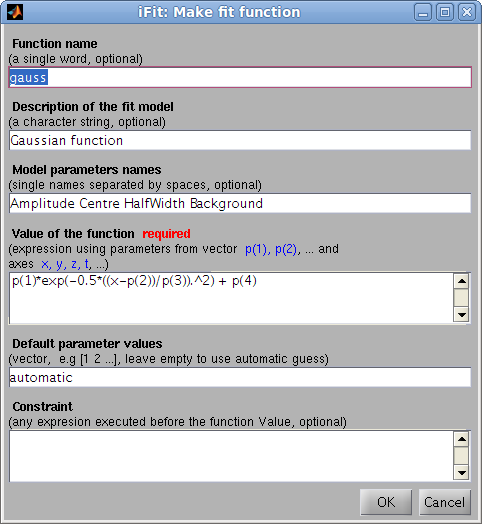expression as a function of the parameter vector p and the axes x,y,z,t,u,v,w
`>> h=ifitmakefunc;>> h=edit(iFunc);	% equivalent`
The only required argument is the expression, but you may as well  enter a function name and description, parameter names and default values, as well as a constraint to be evaluated before computing the function value. When started without any parameters, the Gaussian function settings are used.

The function both creates a function file, and returns its model object, that may evaluate and adjust to a data set with fits.
`>> [p,criteria,message,output]= fits(a, h);>> [p,criteria,message,output]= fits(h, a); % same as above`
A quick definition, without using the dialog, can be performed with the syntax:
`>> h=ifitmakefunc('p(1)*exp( (x-p(2))/p(3) )');>> fits(a,  iFunc('p(1)*exp( (x-p(2))/p(3) )')); % same as above`
where  p is the vector that holds parameters. Axes are x (rows),y (columns),z (pages),t (time). Beware to use a model expression with element-wise division and multiplication operators (./ and .*). The function builder is known to work well for 1D and 2D functions.

You can also build complex models from simpler ones using iFunc methods/operators, such as in
```>> h = gauss + lorz>> h = convn(lorz, 3)>> h = convn(gauss, lorz)>> h = gauss .* lorz; % a 1D model>> h = gauss  * lorz; % a 2D model
```
The full syntax for the model builder is:
`>> h=ifitmakefunc('expression');>> h=ifitmakefunc(iFunc model);	>> h=edit(iFunc model);		% same as above>> h=ifitmakefunc('function_name', 'description', 'Parameter1 Parameter2 ...', ...	'expression', [default parameter values], 'constraint');`
which is equivalent to:
`>> fun.Name='function short name';>> fun.Description='function description';		% function long description>> fun.Parameters='Parameter1 Parameter2 ...';		% parameter names. When empty, names are given according to the Expression analysis (when appropriate)>> fun.Guess=[0 1 2 ...] or 'automatic';		% parameter default values (vector), or automatic mode>> fun.Expression='expression using p and x,y,z,t...';	% value of the model (required). p is the vector that holds parameters. Axes are x,y,z,t,u,v,w.>> fun.Constraint='evaluated before Expression';	% constraint evaluated prior to the model Expression>> h=iFunc(fun);>> fits(a, h);`
The generated code will basically be:
`function y=Name(p, axes, ...)% Description% Parameters: [fun.Parameters]Constraint;y=Expression;`
The resulting function has the ability to identify itself (disp(model) provides detailed informations), compute automatic starting parameters with e.g. feval(model, 'guess'), display itself plot(model), and evaluate its value of course feval(model, parameter_values, axes, ...). The resulting model is a iFunc object. Refer to that object description for more information.

A large set of 1D (vector) and 2D (surface) models can be obtained from ZunZun.com.

#### Creating complex functions from simple functions

To assemble existing functions into new ones, you may use the iFunc operators e.g.
`>> h=gauss+lorz; h.Constraint = 'p(8)=0';`
which creates a new function which is the sum of a Gaussian and a Lorentzian.  Unspecified arguments are guessed/automatically set. The second redundant Lorentzian Background p(8) parameter is forced to 0 so that it does not correlate with the Gaussian Background p(4).

#### Using signal convolution/correlation in new functions

It is possible to include a convolution (FFT based, multidimensional) in the core of the new function. The two function fconv and fconvn should then be used with the following syntax, where a and b are vectors/matrices:
`>> c = fconv(a,b);		% returned convoluted object with size which is size(a)+size(b)+1>> c = fconv(a,b, 'same');	% returned convoluted object with size which is size(a)>> c = fconv(a,b, 'valid');	% returned convoluted object with size which is size(a)-size(b)+1>> c = fconv(a,b, 'pad');	% pads 'a' with starting/ending values to minimize border effects>> c = fconv(a,b, 'center');	% centers 'b' so that convolution does not shift 'a'>> c = fconv(a,b, 'norm');	% normalizes 'b' so that convolution does not change 'a' integral>> c = fconv(a,b, 'background');% subtracts minimal value in 'b' so that convolution does not change 'a' integral>> c = fconv(a,b, 'deconv');	% deconvolution, but very sensitive to noise (use with caution)`
When some data has to be convoluted with a response function (e.g. instrument resolution function), the usual options to use should be:
`>> c = fconv(a,b, 'same pad background center norm');>> c = fconvn(a,b);	% same as above in a shorter call`
These convolution methods have been ported to iFunc models as
• conv: convolution (calls fconv)
• convn: normalized convolution (calls fconvn)
• xcorr: cross-correlation (calls fconv in correlation mode)
If you wish to convolute two models, use
`>> a = convn(lorz, 3)		% convolution of a Lorentzian with a Gaussian of width 3>> a = convn(lorz, gauss)	% a Voigt function...>> a = convn(lorz, 'double(b)'); a.Constraint = 'global b';	% convolute with a global variable 'b'`
where the vector/matrix 'b' holds the response function (filter) with the same axis binning as the object 'a'.

Warning: The fconv and fxcorr functions require the x axis not to be coarsely sampled. In case the models/data contain narrow peaks (described with few points underneath e.g. less than 5), the fit model above will fail convolving. One solution is to artificially create a finer sampled axis, evaluate the function, and reduce back to the initial number of points. The constraints are used to define the new finer axis xx, and the final result is re-sampled with 1 point out of 5 :
`>> h = convn(dho, gauss); >> h.Constraint='x=linspace(min(x),max(x), 5*length(x))';	% artificially extend the 'x' axis>> h = h + 'signal=signal(1:5:end);';				% shrink 'signal' back to initial size`

### How to write manually a model function

We suggest that you start from the 'gauss' function in iFit/Scripts/Models, and customize at will. Please retain the function core structure to ensure proper execution. More complex models can be derived from e.g. the voigt function.
`>> edit gauss>> edit voigt>> ifitmakefunc(voigt)	% pop-up a dialogue>> edit(voigt)		% same as ifitmakefunc for iFunc objects`

E. Farhi - iFit/fit models - Nov. 27, 2018 2.0.2 - back to Main iFit Page